Next: A Simple Example With Up: CHIRPLET TRANSFORM SUBSPACES Previous: CHIRPLET TRANSFORM SUBSPACES

## The Frequency-Frequency (FF) Plane

We begin by discussing the CF plane, and then present an argument for re-parameterizing this plane in terms of two frequency indices, leading to what we will be calling ``frequency-frequency'' (FF) analysis.

Consider a two-dimensional slice through the five-dimensional CCT parameter space that we defined in (11):

S_c,f_c = C_0,f_c,0,c,0 g(t) \: \: s(t)

where s(t) is an arbitrary time series, and the two dimensions of the transform space are the slope of the frequency rise, c, and the center frequency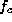. This transform is knownmannVI91 as the ``bowtie (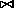) subspace'' since the CF plane of a chirp is a sharp peak surrouned by faint bowtie-shaped contours (Fig. 6). Computing the CF plane of a signal, s(t), is equivalent to correlating the signal with a family of chirps that are parameterized by chirprate, c and center frequency,. Calculating the CF plane from a signal that contains pure tones results in peaks on the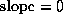axis. Downchirps in the signal result in peaks to the left of this line, and upchirps result in peaks to the right.

Figure: FIGURE GOES SOMEWHERE IN THIS GENERAL VICINITY

For a discrete function, we would have periodicity in the CF plane, and the Nyquist boundary is diamond () shaped. The Nyquist limit dictates that the chirps with the highest (lowest) c values begin with a fractional frequency of -1/2 (+1/2) and end with a frequency of +1/2 (-1/2). These chirps will both lie on the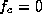axis of the CF plane. Consider a chirp that begins with a frequency 1/4 and ends with a frequency of 3/4. It has the same chirprate: c = 3/4-1/4 = 1/2, but it will violate the Nyquist limit because part of the chirp exceeds the fractional frequency of 1/2, and will therefore give rise to aliasing.

Ideally we would like this transform to have nice rectangular boundaries for convenient viewing on a video display, so we overcome the Nyquist problem by tilting the parameter space. The new chirplets are then given by:

C_0, (f_end+f_beg)/2, 0, (f_end-f_beg)/2, 0 g(t)
= g(t) e^j 2 (f_end-f_beg2t + f_end+f_beg2)t

where g denotes the mother chirplet. The change of coordinates from the CF plane to the FF plane is given byand. When the analysis interval (window) is of finite duration,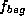may be taken to be the instantaneous frequency of the chirp at the beginning of the analysis interval (time window) and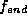the instantaneous frequency at the end of this interval. Since the new parameterization involves two frequency coordinates, we will refer to the resulting parameter space as the ``frequency-frequency'' (FF) plane. Figure 7

Figure: FIGURE GOES SOMEWHERE IN THIS GENERAL VICINITY

shows the FF plane computed from a harmonic oscillation.

The value of the function defined on the FF plane, evaluated at the origin gives a measure of how strong the chirp component from 0 to 0 (the DC component) is. The value at coordinates (0,1/2), for example, gives the strength of the component of a chirp going from a frequency of 0 to 1/2. Values of the FF plane in the upper left half (above and to the left of the diagonal) correspond to upchirps; those to the lower right correspond to downchirps. The values of the FF plane along the diagonal line,, define the Fourier transform of the original time-domain signal; the windowed version of the signal may be entirely re-constructed from only the diagonal of the complex-valued FF plane.Next: A Simple Example With Up: CHIRPLET TRANSFORM SUBSPACES Previous: CHIRPLET TRANSFORM SUBSPACES

Steve Mann
Thu Jan 8 19:50:27 EST 1998